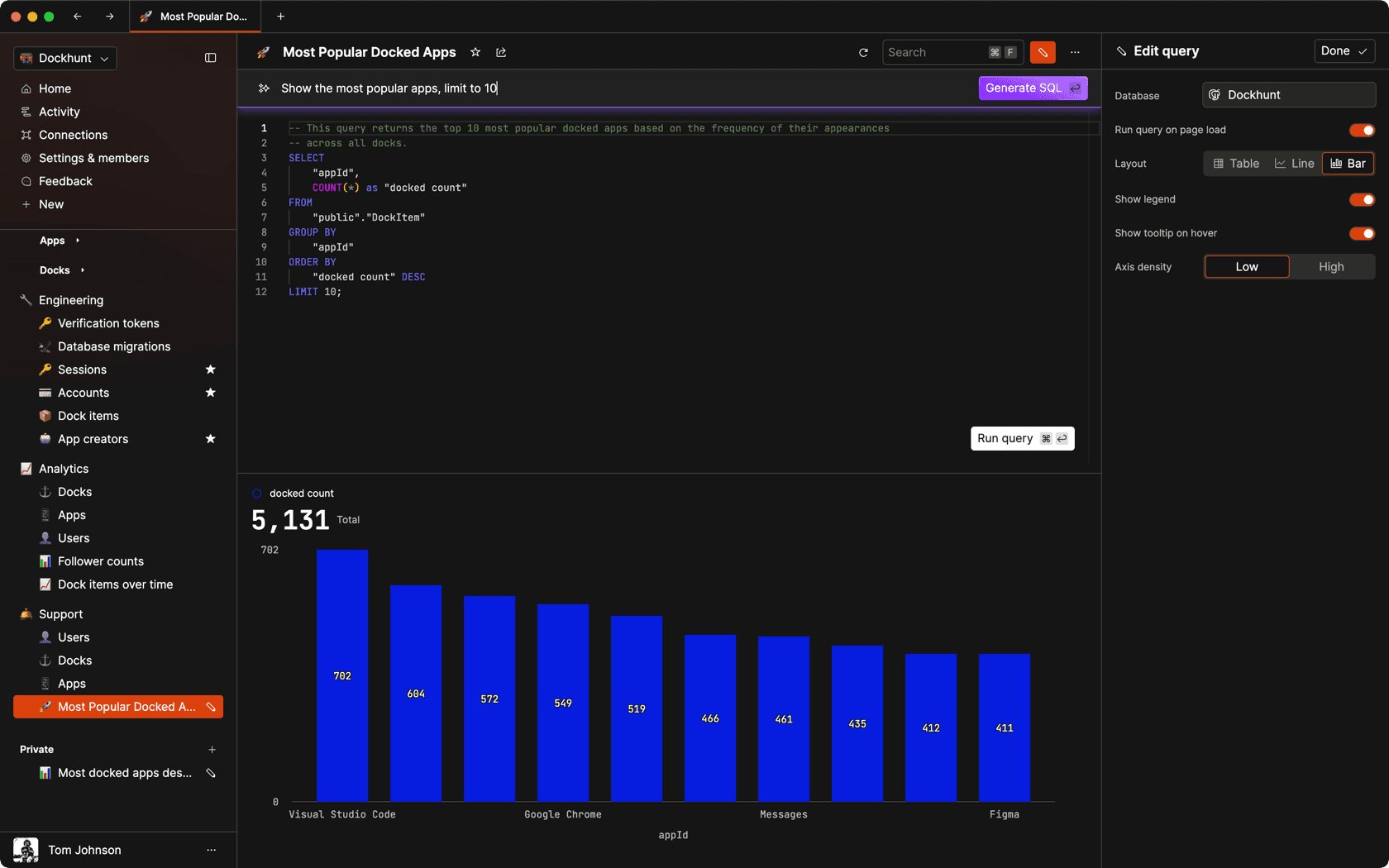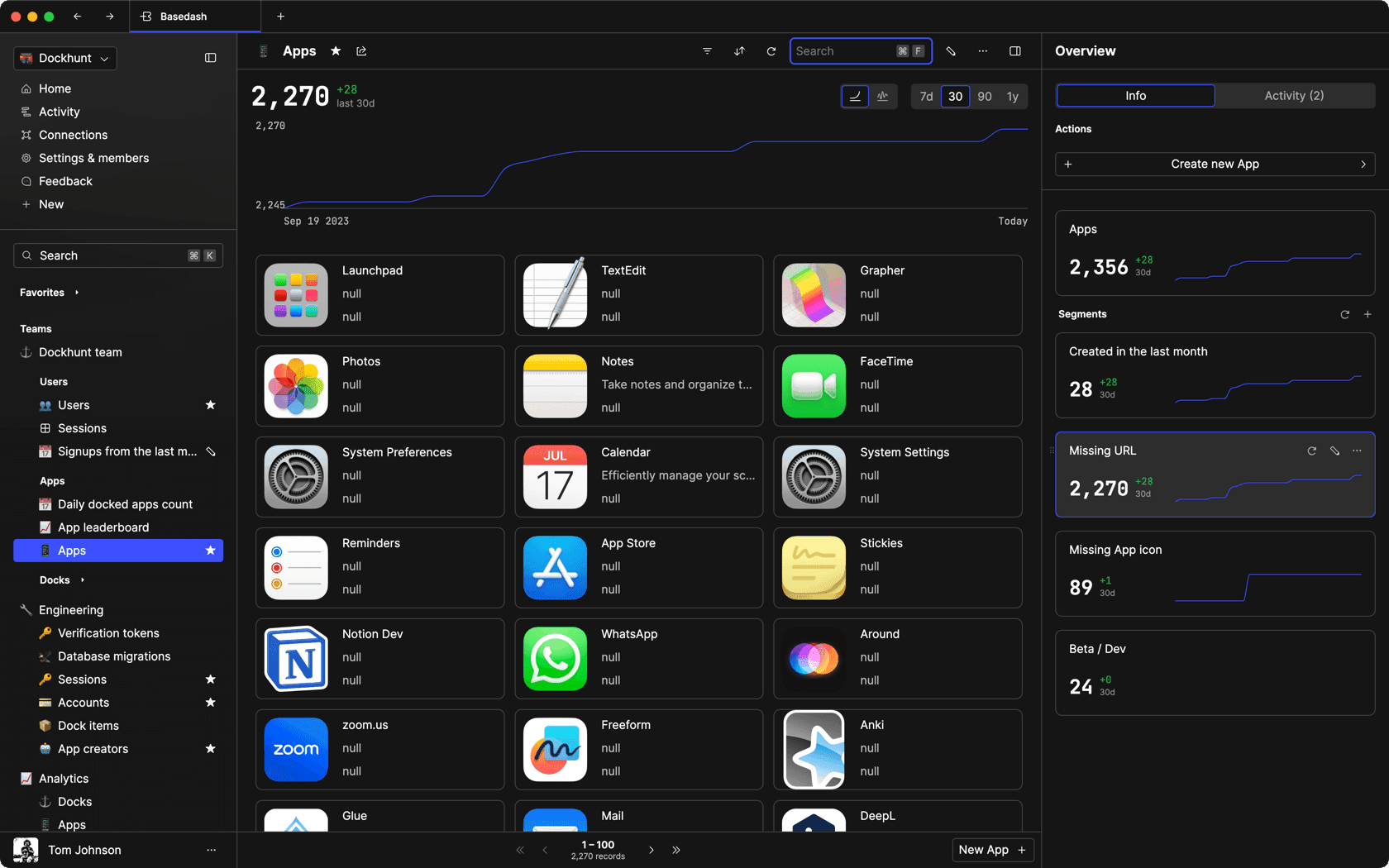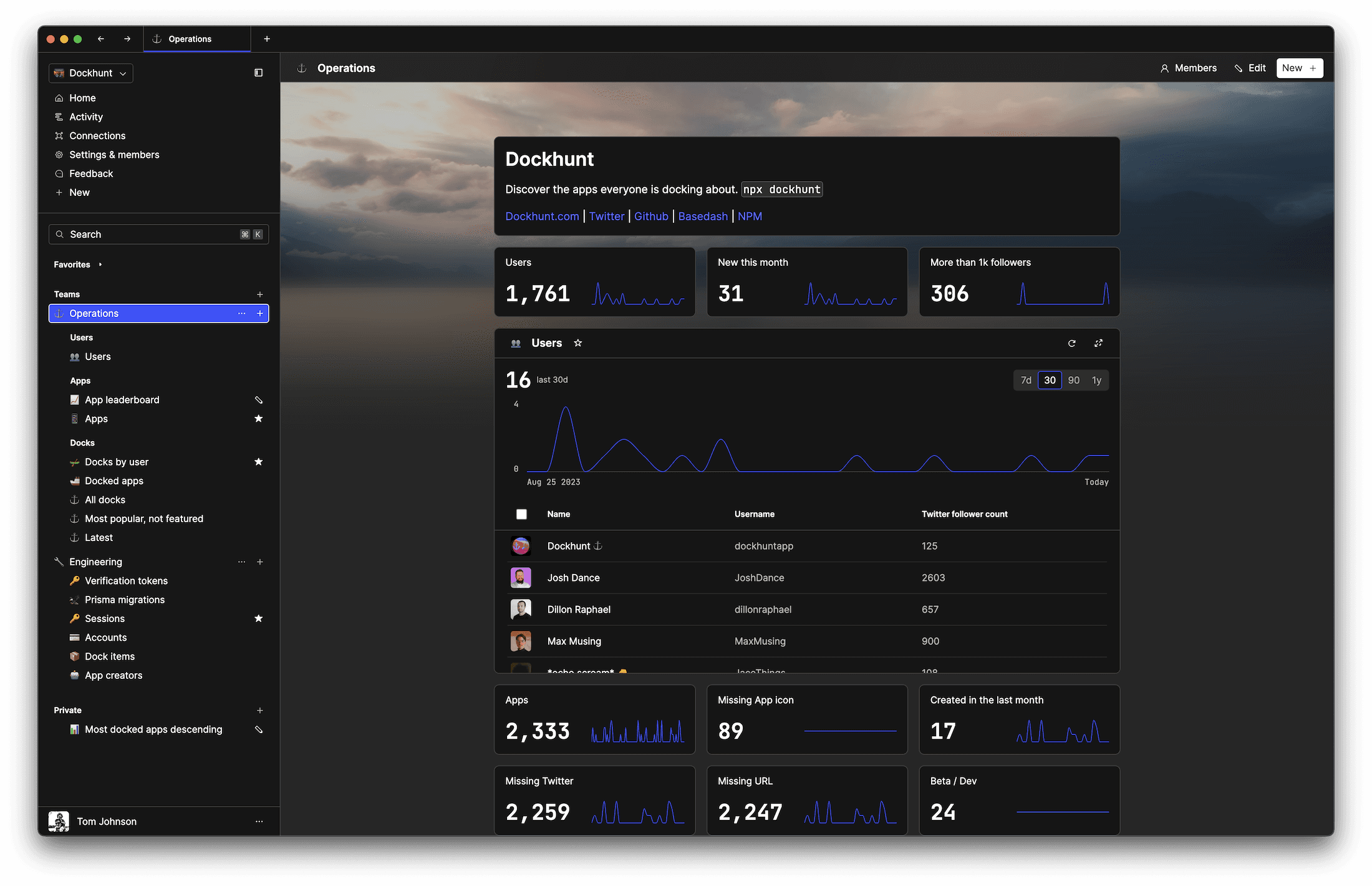# How to Check if a Number is a Float in JavaScriptself.__wrap_n=self.__wrap_n||(self.CSS&&CSS.supports("text-wrap","balance")?1:2);self.__wrap_b=(e,r,t)=>{t=t||document.querySelector(`[data-br="\${e}"]`);let o=t.parentElement,a=s=>t.style.maxWidth=s+"px";t.style.maxWidth="";let n,i=o.clientWidth,l=o.clientHeight,c=i/2-.25,g=i+.5;if(i){for(a(c),c=Math.max(t.scrollWidth,c);c+1<g;)n=Math.round((c+g)/2),a(n),o.clientHeight===l?g=n:c=n;a(g*r+i*(1-r))}t.__wrap_o||typeof ResizeObserver<"u"&&(t.__wrap_o=new ResizeObserver(()=>{self.__wrap_b(0,+t.dataset.brr,t)})).observe(o)};self.__wrap_n!=1&&self.__wrap_b(":R2a9m:",1)

November 8, 2023

In order to determine if a number if a float in JavaScript, you need to understandthe nature of number representation in the language. Since JavaScript uses IEEE 754 standard for floating-point arithmetic, integers and floats are not distinct types. But there are techniques to differentiate them. We’ll cover them below.

## Understanding number representation in JavaScript

In JavaScript, numbers are represented as double-precision 64-bit binary format IEEE 754 values, which means that both integers and floating-point numbers are treated as the same type. This can make it challenging to determine if a number is an integer or a float, as they are not inherently distinguished.

## Using the `Number` object and `parseInt`

The `Number` object provides methods that can be used to inspect the characteristics of numeric values. One approach to check if a number is a float is to compare it with its integer part obtained using `parseInt`.

```function isFloat(n) {
return Number(n) === n && n % 1 !== 0;
}```

## Leveraging `parseFloat` and strict comparison

Alternatively, `parseFloat` can be used to ensure the number remains the same when its floating-point representation is parsed from a string. Strict comparison to the original number then reveals its type.

```function isFloat(n) {
return parseFloat(n) === n && !Number.isInteger(n);
}```

## Utilizing `Math.floor` or `Math.ceil`

Mathematical functions like `Math.floor` or `Math.ceil` can also be used to check for float numbers by comparing the original number with the result of the floor or ceil operation.

```function isFloat(n) {
return Math.floor(n) !== n;
}```

## Employing `Number.isInteger`

A straightforward method provided by ES6 is `Number.isInteger`, which directly checks if a number is an integer, hence its negation would imply a float.

```function isFloat(n) {
return !Number.isInteger(n);
}```

Ship faster, worry less with Basedash

You're busy enough with product work to be weighed down building, maintaining, scoping and developing internal apps and admin panels. Forget all of that, and give your team the admin panel that you don't have to build. Launch in less time than it takes to run a standup.

You're busy enough with product work to be weighed down building, maintaining, scoping and developing internal apps and admin panels. Forget all of that, and give your team the admin panel that you don't have to build. Launch in less time than it takes to run a standup.

You're busy enough with product work to be weighed down building, maintaining, scoping and developing internal apps and admin panels. Forget all of that, and give your team the admin panel that you don't have to build. Launch in less time than it takes to run a standup.### Dashboards and charts

Effortlessly make charts and create a space for your team to work together towards shared goals and metrics.

### SQL composer with AISOC II
Type 2 Certified

### Features

Proudly carbon-neutral.SOC II
Type 2 Certified

### Features

Proudly carbon-neutral.SOC II
Type 2 Certified